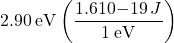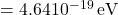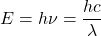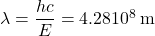## PLEASE HELP ME WITH THIS ONE QUESTION A photon has 2.90 eV of energy. What is the photon’s wavelength? (h = 6.626 x 10^-19, 1 eV = 1.

Question

A photon has 2.90 eV of energy. What is the photon’s wavelength? (h = 6.626 x 10^-19, 1 eV = 1.6 x 10^-19 J)

in progress 0
6 months 2021-07-13T15:56:03+00:00 1 Answers 5 views 0

1. Explanation:

First, we convert the energy from eV to Joules:We know from definition thatso the wavelength of the photon is